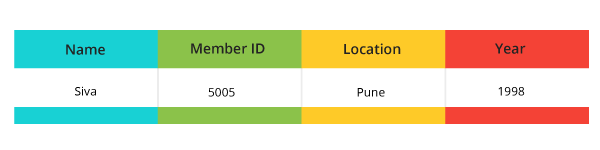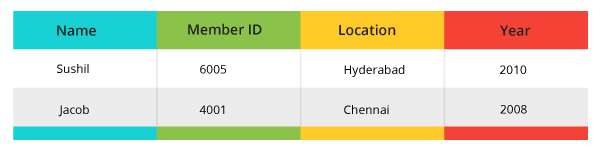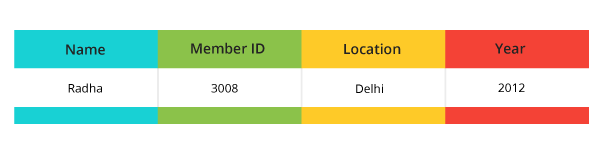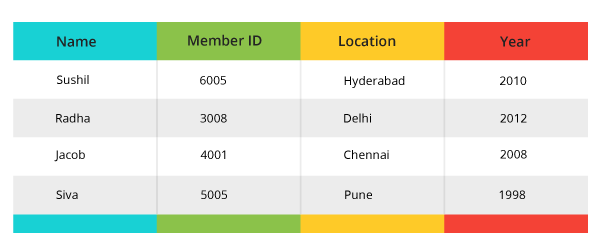Special Offer - Enroll Now and Get 2 Course at ₹25000/- Only Explore Now!# SQL Where Clause

December 16th, 2019

## SQL Where Clause

In this Blog you will learn how to Fetch Filter Data from Database Tables using SQL Where Clause and also learn to specify a condition while fetching the data from a single table or by joining with multiple tables.

WHERE clause

A where condition is optional part in SELECT and UPDATE & DELETE statements.
In the Where clause let you selected the rows based on the Boolean expression. If the expression is “TRUE” then results will be returned.

Note: WHERE clause return after the Oracle FROM clause.

Here we can use the operators in where the condition

### Basic operators :

• =( EQUAL), <(LESS THEN)
• => (GREATER THEN EQUAL)
• =< (LESS THEN EQUAL)
• <> (NOT EQUAL)

• IN
• ALL
• BETWEEN
• LIKE
• AND
• OR

WHERE clause can be used in JOINS also Like

• INNER JOIN
• OUTER JOIN
• LEFT JOIN
• RIGHT JOIN

It can be used in DATE Range

#### Syntax:

Select * from table name WHERE column_name operational value.

#### Example:

Postal table with the data.
Select   *   from postal;

### SELECT Statement

Select  *  from postal where MemberID=5005;

#### Output:Select   *   from postal where MemberID IN (6005, 4001);

####Select   *   from postal where Location LIKE ’%Del%’;

#### Output:### UPDATE Statement

• Update postal

Set location=’Mumbai’
Where Member ID=’5005’;
Commit;

• Select *  from postal where MemberID=5005;

Output:

Delete from postal

Where Name= ‘Ravi’;

Commit;